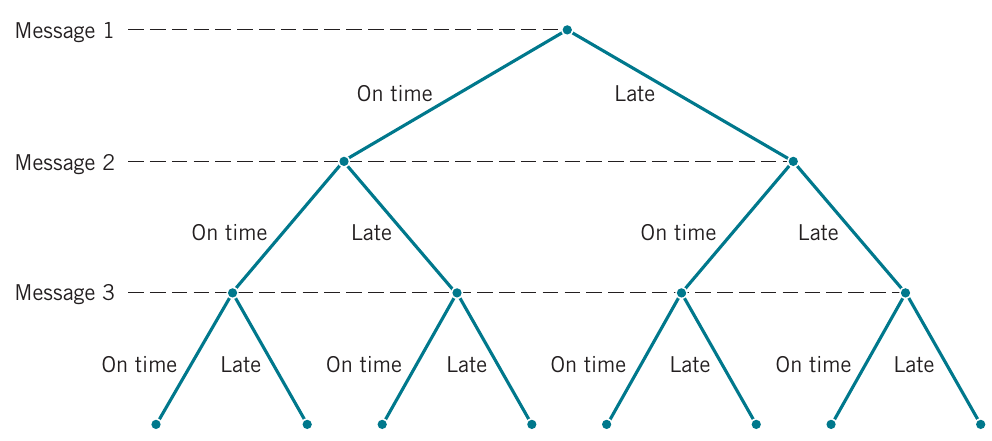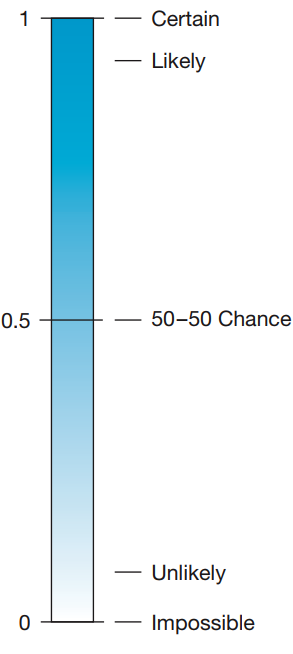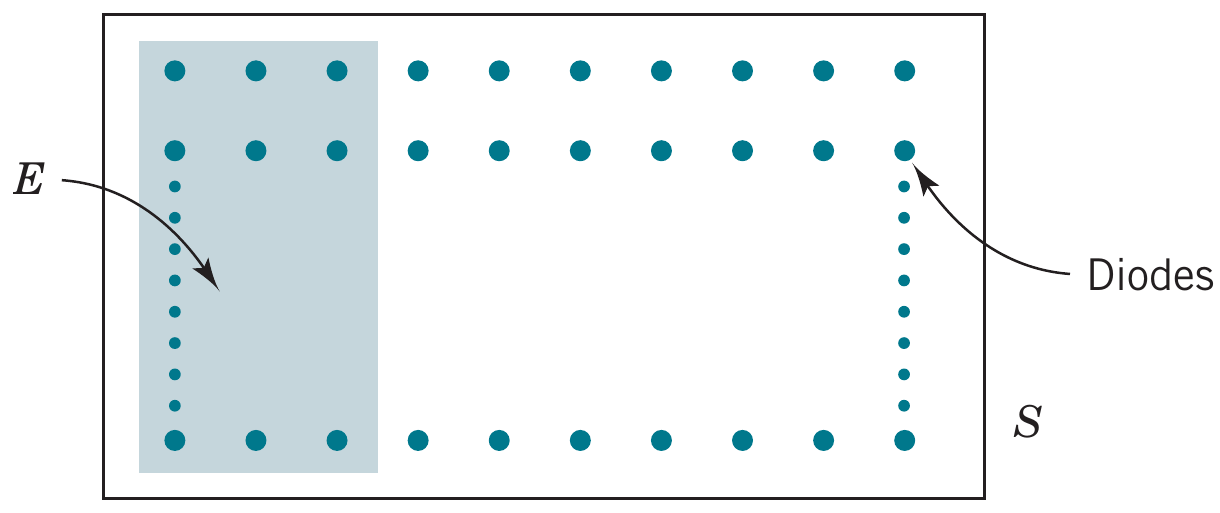# Counting

02/01/2021

## Instructions:

Use the left and right arrow keys to navigate the presentation forward and backward respectively. You can also use the arrows at the bottom right of the screen to navigate with a mouse.

FAIR USE ACT DISCLAIMER:
This site is for educational purposes only. This website may contain copyrighted material, the use of which has not been specifically authorized by the copyright holders. The material is made available on this website as a way to advance teaching, and copyright-protected materials are used to the extent necessary to make this class function in a distance learning environment. The Fair Use Copyright Disclaimer is under section 107 of the Copyright Act of 1976, allowance is made for “fair use” for purposes such as criticism, comment, news reporting, teaching, scholarship, education and research.

## Outline

• The following topics will be covered in this lecture:

• Counting techniques
• Permutations and combinations
• Interpretations of probability

## Counting Techniques

• Determining the outcomes in the sample space (or an event) is not always straight forward
• Recall the example of messages with the tree diagramCourtesy of Montgomery & Runger, Applied Statistics and Probability for Engineers, 7th edition

• The size of the sample space equals the number of branches in the last level of the tree, and this quantity equals $2\times 2 \times 2 = 8$
• Note that this can also be counted by looking at the number of choices we have at each level of the tree, given the number of previous choices.
• That is to say, we have two ways of making the first choice “On time” or “Late”.
• Given the previous choice, the next level has two more choices.
• Finally, given the previous two choices, the next level has two more choices.

### Multiplication rule

• The previous idea can be formalized as follows.
• Multiplication Rule (for counting techniques)
Assume an operation can be described as a sequence of $$k$$ steps, and
• $$n_1$$ is the number of ways to complete step 1
• $$n_2$$ is the number of ways to complete step 2 for each way to complete step 1, and
• $$n_3$$ is the number of ways to complete step 3 for each way to complete step 2, and
• so forth.
• Then the total number of ways to complete the operation is $n_1\times n_2\times \dots \times n_k$
• EXAMPLE: consider the design for a Website consists of four colors, three fonts, and three positions for an image.
• We can first choose one of $$n_1=4$$ colors.
• Given any choice of a color, there are $$n_2=3$$ choices of fonts.
• Given any choice of color and font, there are $$n_3=3$$ positions.
• From the multiplication rule, $$4\times 3 \times 3 = 36$$ different designs are possible.

### Permutations

• A permutation of the elements is an ordered sequence of the elements.
• For example, considering the set with elements $$S=\{a,b,c\}$$
• $$abc, acb, bac, bca, cab,$$ and $$cba$$ are all of the permutations of the elements of $$S$$.
• From the multiplication rule we have
• The number of permutations of $$n$$ different elements is $$n!$$ where $n! = n\times(n-1)\times(n-2)\times \dots \times 2 \times 1$
• This is understood as:
• there are $$n$$ total choices for the first position;
• subsequently, we have reduced our choices by 1;
• therefore there are $$n-1$$ choices for the second position given a choice of the first.
• Having chosen the first two positions, there remain $$n-2$$ choices for the third position;
• and so on…

### Permutations of subsets

• We may also be interested in the number of arrangements of only some of the elements of a set.
• From the multiplication rule we also have
• The number of permutations of subsets of $$r$$ elements selected from a set of $$n$$ different elements is $P_r^n = n\times(n-1)\times(n-2)\times\dots\times(n-r+1)=\frac{n!}{(n-r)!}$
• This follows the identical argument as before, but where we now divide out by the trailing terms for the choices in positions beyond $$n-r$$.
• EXAMPLE: a printed circuit board has eight different locations in which a component can be placed.
• Q: If four different components are to be placed on the board, how many different designs are possible?
• Each design consists of first selecting a location from the eight locations for the first component.
• Subsequently, we select a location from the remaining seven for the second component.
• Then we select a location from the remaining six for the third component.
• Finally, we select a location from the remaining five for the fourth component.
• At this point, there are no more remaining placements to be made, as we were only placing four components.
• Therefore, we can count equivalently, $8\times 7 \times 6 \times 5 = \frac{8!}{4 \times 3 \times 2 \times 1} = \frac{8!}{4!} = P_4^8,$ dividing out by the trailing terms for the positions beyond $$n-r$$.
• We find $$P_4^8=8\times 7 \times 6 \times 5=\frac{8!}{4!} = 1680$$

### Permutations of similar objects

• If we are interested in counting the number of ordered sequences for objects that are not all different
• The number of permutations of $$n = n_1 + n_2 +\dots + n_r$$ objects of which $$n_1$$ are of one type, $$n_2$$ are of a second type, … , and $$n_r$$ are of an $$r$$-th type is $\frac{n!}{n_1!n_2!n_3!\dots n_r!}$
• This version of the rule accounts for the redundancy in counting non-different objects multiple times.
• We divide out by the number of ways to permute identical objects from a given class of objects, of class-size $$n_i$$.
• EXAMPLE: a hospital operating room needs to schedule three knee surgeries and two hip surgeries in a day.
• In this context, $$n= 3 + 2$$ where $$n_1=3$$ is the number of knee surgeries and $$n_2 =2$$ is the number of hip surgeries.
• Therefore, the number of possible sequences of three knee and two hip surgeries is $\frac{5!}{2!3!}=10$
• If we denote a knee and hip surgery as $$k$$ and $$h$$, respectively.
• the actual permutations are all ways we can maked ordered arrangements of three of the letter $$k$$ and two of the letter $$h$$: $\{kkkhh, kkhkh, kkhhk, khkkh, khkhk, khhkk, hkkkh, hkkhk, hkhkk, hhkkk\}$

### Combinations

• Another counting problem of interest is the number of subsets of $$r$$ elements that can be selected from a set of $$n$$ elements.
• For such a counting problem, order is not important.
• This differs from a schedule as before, where the order matters.
• The number of combinations, subsets of $$r$$ elements that can be selected from a set of $$n$$ elements, is denoted as $$C_r^n$$ or $$\binom{n}{r}$$ $C_r^n = \binom{n}{r}= \frac{n!}{r!(n-r)!}$
• Recall, we find permutations of $$r$$ objects from a collection of size $$n$$ as $$P^n_r = \frac{n!}{\left(n-r\right)!}$$.
• The above formula corresponds to when we consider the order to matter;
• however, if we count all arrangements as being identical, we divide out by the total ways to permute the arrangments of size $$r$$ to avoid multiple counting.
• EXAMPLE: a printed circuit board has eight different locations in which a component can be placed.
• Q: If five identical components are to be placed on the board, how many different designs are possible?
• Each design is a subset of size five from the eight locations that are to contain the components.
• The order of the components doesn’t matter, because all components are identical.
• From the equation above, the number of possible designs is $C_5^8 = \binom{8}{5}= \frac{8!}{5!3!}=56.$

### Sampling with or without replacement

• In random experiments in which items are selected from a batch, an item may or may not be replaced before the next one is selected
• This is referred to as sampling with or without replacement
• EXAMPLE: a bin of 50 manufactured parts contains 3 defective parts and 47 non-defective parts.
• A sample of 6 parts is selected without replacement – that is, each part can be selected only once, and the sample is a subset of the 50 parts.
• Q: How many different samples are there of size 6 that contain exactly 2 defective parts?
• A subset containing exactly 2 defective parts can be formed by first choosing the 2 defective parts from the 3 defective parts.
• Using the equation for combinations, the number of different ways this first step can be completed is $\binom{3}{2}= \frac{3!}{2!1!}$
• Then, the second step is to select the remaining 4 parts from the 47 acceptable parts in the bin. The number of different ways the second step can be completed is $\binom{47}{4}= \frac{47!}{4!43!}=178,365$
• From the multiplication rule, the number of subsets of size 6 that contain exactly 2 defective parts is $3\times 178,365 = 535, 095$

## Probability for discrete sample spaces

• Counting rules like the previous ones form the basis for computing simple probabilities.

• The classical model for probability is based on a finite, discrete sample space where all simple events are equally likely.

• For example, we might consider drawing cards randomly from a standard deck of cards.
• We will assume that drawing any card is as likely as drawing any other – drawing any given card will be considered a simple event.
• This situation occurs (approximately) if we assume that the deck is well-shuffled and we draw a card from the top of the deck.
• We know that half of the cards are black and half of the cards are red.

• If we want to estimate the probability of drawing two black cards, we can use a simple counting argument to find all the ways for this to occur:

$26 \times 25$

given each choice, sampling without replacement.

• We can also count all possible ways to draw any two cards:

$52 \times 51.$

### Probability for discrete sample spaces – continued

• Considering the number of ways to select two black cards, relative to the number of ways to select any two cards, we compute our simple probability model as, $\text{Probability of selecting two black cards} = \frac{26 \times 25}{52 \times 51}.$
• Probability is used to quantify the likelihood, or chance, that an outcome of a random experiment will occur.Courtesy of Mario Triola, Essentials of Statistics, 6th edition

• The likelihood of an outcome is quantified by assigning a number from the interval $$[0, 1]$$ to the outcome (or a percentage from 0 to 100%).
• Higher numbers indicate that the outcome is more likely than lower numbers.
• A probability of $$0$$ indicates an outcome will not occur.
• A probability of $$1$$ indicates that an outcome will occur with certainty.

## Equally Likely Outcomes

• Probabilities for a random experiment are often assigned on the basis of a reasonable model of the system under study.
• One approach already mentioned is to base probability assignments on the simple concept of equally likely outcomes.
• Equally Likely Outcomes:
Whenever a sample space consists of $$N$$ possible outcomes that are equally likely, the probability of each outcome is $$1/N$$.
• EXAMPLE: rolling a fair six-sided die, we can compute this directly.
• We know exactly all possible simple events (rolling $$1$$ through $$6$$)
• With all sides equally likely, the probability of rolling any number is $$\frac{1}{6}$$.
• EXAMPLE: Suppose that we select 1 laser diode randomly from a batch of 100.Courtesy of Montgomery & Runger, Applied Statistics and Probability for Engineers, 7th edition

• By randomly we meam that it is reasonable to assume that each diode in the batch has an equal chance of being selected.
• The probability model for this experiment assigns probability of $$\frac{1}{100}=0.01$$ to each of the 100 outcomes
• The probability of an event is determined as the ratio of the number of outcomes in the event to the number of outcomes in the sample space (for equally likely outcomes).

## Relative frequency interpretation of probability

• The classical approach to probability works mostly for cases like games of chance, where the the sample space is limited and all outcomes are equally likely.
• Another interpretation of probability is based on the conceptual model of repeated replications of the random experiment.
• The probability of an outcome is interpreted as the limiting value of the proportion of times the outcome occurs in $$n$$ repetitions of the random experiment as $$n$$ increases beyond all bounds.
• However, many examples cannot be broken into equally likely simple events.
• A simple counter-example is an unfair, weighted coin.
• We cannot accurately calculate the probability to flip a “heads” with a simple counting argument when the coin has been weighted to come up on heads more often.
• If we don’t know how the coin is weighted, a natural way to approximate the true probability is by replication of the process.
• For example, we can flip the coin and record whether it lands on heads or tails.
• Let $$A=$$"coin lands heads" and $$B=$$"coin lands tails".
• We will construct a frequency distribution for both of these events over many repeated flips.
• We will then approximate the true probability of event $$A$$ by the frequency of $$A$$ relative to all replications of the process.
• This is a natural way of thinking about probability – if we could flip a coin infinitely many times we could get the true value in some limiting average.

## Probability of an event

• For general probabilities (not necessarily equally-likely simple events), we can define the probability of an event as follows.
• Probability of an event
For a discrete sample space, the probability of an event $$E$$, denoted as $$P(E)$$, equals the sum of the probabilities of the outcomes in $$E$$.
• EXAMPLE: a random experiment can result in one of the outcomes $$\{a, b, c, d\}$$ with probabilities $$0.1, 0.3, 0.5,$$ and $$0.1$$, respectively. Let $$A$$ denote the event $$\{a, b\}$$, $$B$$ the event $$\{b, c, d\}$$, and $$C$$ the event $$\{d\}$$
• Question: what is $$P(C)?$$
• Since the event $$C$$ is $$\{d\}$$ only considers one outcome, the probability is given as $$P(C)=0.1$$
• Question: what is $$P(B)?$$
• Since the event $$B$$ is $$\{b,c,d\}$$, we must consider the three possible outcomes:
• $$\{b\}$$ with probabilty $$0.3$$, $$\{c\}$$ with probabilty $$0.5$$, and $$\{d\}$$ with probabilty $$0.1$$
• Sum the probabilities of the different outcomes $$P(B) = 0.3 + 0.5+0.1 = 0.9$$
• EXAMPLE: if now we want to compute the probability of rolling an even number on a fair, six-sided die
• Question: how many ways we can roll an even number?
• The outcomes can be $$2,4,6,$$ so three ways, and with all sides equally likely, the chance is $\frac{1}{6}+\frac{1}{6}+\frac{1}{6}=\frac{3}{6}=0.5$
• This can also be computed with a similar counting argument to earlier, noting that half of the sides are even.

## Probability of an event – sampling without replacement example

• Recall the inspection example described earlier. From a bin of 50 parts, 6 parts are selected randomly without replacement. The bin contains 3 defective parts and 47 nondefective parts.
• Question: what is the probability that exactly 2 defective parts are selected in the sample?

• The sample space consists of all possible (unordered) subsets of 6 parts selected without replacement $$\binom{3}{2} \times \binom{47}{4}=535,095$$ (from earlier)
• where the total number of different subsets of size 6 is $$\binom{50}{6}=15,890,700$$
• Since the probability is determined as the ratio of the number of outcomes in the event to the number of outcomes in the sample space (for equally likely outcomes).
• Therefore, the probability that a sample contains exactly 2 defective parts is $\frac{535,095}{15,890,700}=0.034$